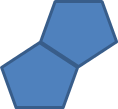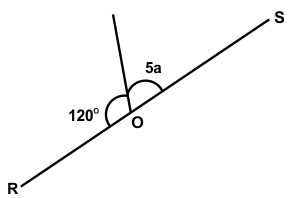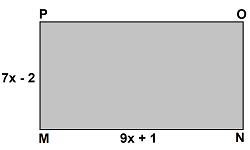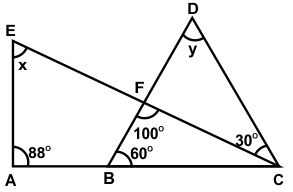﻿ Maths Olympiad (CMO) Sample Paper & Questions for Class 6

# CREST Mathematics Olympiad Class 6 Sample Paper

Sample PDF of CREST Mathematics Olympiad for Class 6:

## Syllabus:

Section 1: Knowing Our Numbers, Whole Numbers, Playing with Numbers, Basic Geometrical Ideas, Understanding Elementary Shapes, Integers, Fractions, Decimals, Data Handling, Mensuration, Algebra, Ratio And Proportion, Symmetry, Practical Geometry.

Achievers Section: Higher Order Thinking Questions - Syllabus as per Section 1

 Q.1 The average marks in Mathematics for 5 students were found to be 50. Later it was discovered that in the case of one student, the mark 48 was misread as 84. The correct average is:
 Q.2 Which of the following statements is true?
 Q.3 Two regular pentagons of side 5 cm are joined together as shown in the figure. Find the perimeter of the new figure:Q.4 Simplify: (87.4 x 6.5 - 0.1) / (28.4 x 9.8 + 5.68)
 Q.5 It takes 90 minutes to wash 20 vehicles at a car wash. At this rate, how many minutes does it take to wash 5 vehicles?
 Q.6 Two numbers are such that the ratio between them is 3:4. If each is increased by 9, the ratio between the new numbers formed is 6:7. Find the original numbers.
 Q.7 Which of the following statements is true?
 Q.8 Find the value of 'a' in the below figure, given that ROS is a straight line.Q.9 In the given rectangle MNOP, if length is decreased by 3x, then find the new perimeter.Q.10 In the figure shown below, ABC and EFC are straight lines. Find the value of x + y.Q.1 c Q.2 c Q.3 b Q.4 c Q.5 c Q.6 d Q.7 d Q.8 c Q.9 c Q.10 d

## Maths Olympiad Questions For Class 6

1. Find the next term in the series given below.
3F, 6G, 11I, 18L, ?

a) 21O
b) 25N
c) 27P
d) 27Q

2. What is the difference between the face and place value of 3 in number 456783?

a) 0
b) 1
c) 2
d) 3

3. The sum of 3 + 3 x 3(4 + 3) is:

a) 38
b) 42
c) 45
d) 66

4. How many prime numbers are there between 10 and 20?

a) 7
b) 4
c) 3
d) 5

5. Which of the following properties is incorrect with respect to the properties of whole numbers?

a) Addition and subtraction are commutative.
b) Division by 0 is not defined.
c) Multiplication is distributive over addition.
d) They are closed under addition and multiplication.

6. Complete the number series given below.

1, 4, 2, 8, 6, 24, 22, 88, (?)

a) 86
b) 352
c) 90
d) 154

7. A bag contains 25-paise and 50-paise coins whose total value is Rs. 30. If the number of 25-paise coins is four times that of 50-paise coins, then find the number of each type of coins?

a) 40 and 30
b) 20 and 40
c) 80 and 20
d) 60 and 20

8. Fill in the blank:

1 million = _______ hundred thousand.

a) One
b) Ten
c) Hundred
d) Twenty

9. Virat can type 28 words per minute. At this rate, how many words can Virat type in 5.5 minutes?

a) 154
b) 157
c) 159
d) 162

10. Convert 5 km into metre.

a) 500 m
b) 50 m
c) 5000 m
d) 5 m

11. The numbers which are multiples of 2 are called -

a) odd
b) even
c) prime
d) composite

12. If ‘+’ means ×, ‘–’ means ÷, ‘×’ means –, and ‘÷’ means +, then 16 ÷ 64 – 8 × 4 + 2 = ?

a) 12
b) 16
c) 18
d) 24

13. Where do we place the positive numbers on a vertical number line with respect to 0?

a) Above
b) One its left side
c) One its right side
d) Below

14. The measurement of an angle is 180?. Which one of the following types of angle is this?

a) Right angle
b) Straight angle
c) Reflex angle
d) All of these

15. A number which is a factor of every number is ___.

a) 0
b) 1
c) 2
d) 5

16. Every number is a ____ and a ____ of itself.

a) factor, multiple
b) prime, composite
c) even, odd
d) none

17. 234 + 456 =456 + 234. Which property is depicted in this sum?

a) Associative
b) Commutative
c) Closure
d) Distributive

18. How many lines of symmetry does a semi-circle have?

a) Two
b) One
c) Three
d) Four

19. Which of the following property cannot be applied for subtraction?

a) Associative
b) Closure
c) Distributive
d) All of the above

20. Find the number which when multiplied by 12 gives 36.

a) 3
b) 8
c) 4
d) 12

21. The additive identity of any whole number is _______.

a) 1
b) 0
c) Number itself
d) None of the above

22. Convert 45/1000 into decimals

a) 0.045
b) 0.450
c) 000.45
d) 0.45

23. Find the LCM of 9, 14, 15, 18, 12.

a) 126
b) 252
c) 630
d) 1260

24. Find the equivalent fraction of 5/7 with denominator 21.

a) 25/21
b) 8/21
c) 15/21
d) 2/21

25. Which of the following is an example of an even number?

a) 10351
b) 20989
c) 69007
d) 973572

26. 47.521 L is equal to:

a) 47521 ml
b) 75214 ml
c) 45217 ml
d) 72514 m

27. Add : 26.102 + 6.33

a) 32.342
b) 86.432
c) 32.432
d) 86.135

28. Product of a number by 3 is equal to 171 then the number is:

a) 53
b) 63
c) 57
d) 97

29. Expanded form of M + DC + LXX + IX = ?

a) 1015
b) 1679
c) 1999
d) 2000

30. Find the value of X in the given expression.
832.58 – 242.31 = 779.84 – X

a) 179.57
b) 199.57
c) 295.05
d) 189.57

31. Which of the following is the nearest ten for ?

a) 70
b) 20
c) 50
d) 60

32. A table-cover measures 2 m 45 cm by 1 m 25 cm. Find the perimeter of the table cover.

a) 6 m 80 cm
b) 7 m 40 cm
c) 8 m 70 cm
d) 7 m 70 cm

33. If '+' means '÷', '×' means '–', '–' means '×' and '÷' means '+' then what is the value of given below statement?

38 + 19 – 16 × 17 ÷ 3

a) 18
b) 17
c) 16
d) 12

34. Which of the following fractions is the greatest? 4/7, 4/9, 4/9, 7/8, 1/3

a) 4/7
b) 7/6
c) 4/9
d) 7/8

35. Solve the following Roman numerals.

LIX + XXXVI + IX + XC – LX

a) CXXXXVI
b) CXXXLIV
c) CXXVII
d) CXXXIV

36. One billion equals

a) 1 Crore
b) 10 Crore
c) 100 Crore
d) 1000 Crore

37. If 2x + 3 = 5, which of the following is the value of ‘x’?

a) -4
b) 4
c) -1
d) 1

38. Fill in the box: 500 + 55 = 400+ [?]

a) 55
b) 100
c) 155
d) 500

39. If the dividend and divisor have unlike signs, what is the sign of the quotient?

a) positive
b) negative
c) zero
d) indeterminate

40. A book of 1,456 pages has 9,95,904 words in it. The number of words in each page is _______.

a) 584
b) 684
c) 675
d) 595

41. Evaluate: 7×|−15|−|−9|×8

a) 22
b) 33
c) 11
d) −120

42. Which of the following number is divisible by 3?

a) 18
b) 60
c) 24
d) All of the above

43. Find the sum of the given expression.

(–172) + (–40) + 5 + (–425) + (–275) + 600 – (–15)

a) 315
b) –21
c) 40
d) –292

44. Complete the series 1, 15, 16, 31, 47, 78, 125, _______

a) 167
b) 139
c) 72
d) 203

45. Choose the correct option:
The HCF and LCM of two numbers are 84 and 21, respectively. If the ratio of the two numbers is 1:4, then the larger of the two numbers is:

a) 12
b) 48
c) 84
d) 108

46. A basket contains 300 mangoes. 75 mangoes were distributed among students. The percentage of mangoes left in the basket is

a) 25%
b) 30%
c) 50%
d) 75%

47. 50 chairs and 30 blackboards were purchased for a school. If each chair costs Rs. 1065 and each blackboard costs Rs. 1645, then find the total amount of the bill:

a) Rs. 102,060
b) Rs. 106,500
c) Rs. 120,650
d) Rs. 102,600

48. The LCM of two numbers is 27. The numbers cannot be____.

a) 3, 27
b) 1, 27
c) 3, 9
d) 9, 27

49. Which of the following fractions is closest to 0 ?

a) 5/12
b) 2/3
c) 5/6
d) 3/4

50. The ratio of two numbers is 3:7. If the sum of the numbers is 700, find the two numbers:

a) 210,490
b) 270, 490
c) 110, 490
d) 110, 650